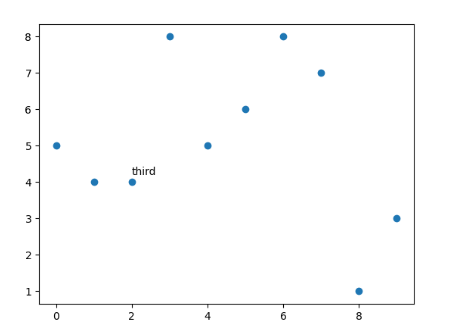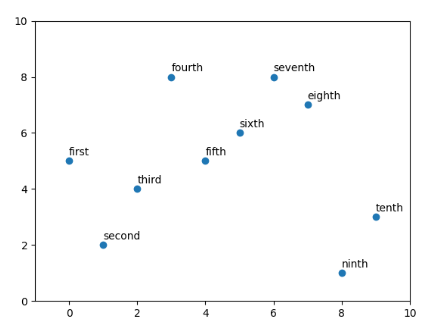GeeksforGeeks App
Open AppBrowser
Continue

# How to Annotate Matplotlib Scatter Plots?

A scatter plot uses dots to represent values for two different numeric variables. In Python, we have a library matplotlib in which there is a function called scatter that helps us to create Scatter Plots. Here, we will use matplotlib.pyplot.scatter() method to plot.

Syntax : matplotlib.pyplot.scatter(x,y)

Parameters:

• x and y are float values and are the necessary parameters to create a scatter plot
• marker : MarkerStyle, default: rcParams[“scatter.marker”] (default: ‘o’)
• cmap : cmapstr or Colormap, default: rcParams[“image.cmap”] (default: ‘viridis’)
• linewidths : float or array-like, default: rcParams[“lines.linewidth”] (default: 1.5)
• alpha : float, default: None → represents the transparency

Annotation of matplotlib means that we want to place a piece of text next to the scatter. There can be two cases depending on the number of the points we have to annotate :

1. Single point annotation
2. All points annotation

### Single Point annotation

In single-point annotation we can use matplotlib.pyplot.text and mention the x coordinate of the scatter point and y coordinate + some factor so that text can be distinctly visible from the plot, and then we have to mention the text.

Syntax: matplotlib.pyplot.text( x, y, s)

Parameters:

• x, y : scalars — The position to place the text. By default, this is in data coordinates. The coordinate system can be changed using the transform parameter.
• s : str — The text.
• fontsize — It is an optional parameter used to set the size of the font to be displayed.

Approach:

1. Import libraries.
2. Create data.
3. Make scatter plot.
4. Apply plt.text() method.

Implementation:

## Python3

 `# Importing libraries``import` `matplotlib.pyplot as plt`` ` `# Preparing dataset``x ``=` `[x ``for` `x ``in` `range``(``10``)]``y ``=` `[``5``, ``4``, ``4``, ``8``, ``5``, ``6``, ``8``, ``7``, ``1``, ``3``]`` ` `# plotting scatter plot``plt.scatter(x, y)`` ` `# annotation of the third point``plt.text(``2``,``4.2``,``"third"``)``plt.show()`

Output:### All points annotation

If we want to annotate all points in the scatter plot then matplotlib.pyplot has an inbuilt function annotate which takes the text, x, and y coordinates of the point.

Syntax: matplotlib.pyplot.annotate( text, xy )

Parameters:

• text : str — The text of the annotation. s is a deprecated synonym for this parameter.
• xy : (float, float) — The point (x, y) to annotate. The coordinate system is determined by xy coordinates.

Approach:

1. Import libraries.
2. Create data.
3. Store all the annotations in a list in order with the sequence of the points to be displayed.
4. Draw the scatter plot.
5. Using a for loop annotate each point.

Implementation:

## Python3

 `# Importing libraries``import` `matplotlib.pyplot as plt`` ` `# Preparing dataset``x ``=` `[x ``for` `x ``in` `range``(``10``)]``y ``=` `[``5``, ``2``, ``4``, ``8``, ``5``, ``6``, ``8``, ``7``, ``1``, ``3``]``text ``=` `[``"first"``, ``"second"``, ``"third"``, ``"fourth"``, ``"fifth"``,``        ``"sixth"``, ``"seventh"``, ``"eighth"``, ``"ninth"``, ``"tenth"``]`` ` `# plotting scatter plot``plt.scatter(x, y)`` ` `# Loop for annotation of all points``for` `i ``in` `range``(``len``(x)):``    ``plt.annotate(text[i], (x[i], y[i] ``+` `0.2``))`` ` `# adjusting the scale of the axes``plt.xlim((``-``1``, ``10``))``plt.ylim((``0``, ``10``))``plt.show()`

Output:My Personal Notes arrow_drop_up